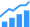Knowledge Center

# Quantum Computing

A different way of processing data using qubits.### Description

The integrated circuit industry has been dealing with quantum phenomena for a long time. A sufficiently small structure can create a quantum well or a quantum dot, trapping charged particles inside. Quantum wells are essential in semiconductor lasers, for example. In silicon integrated circuits, a sufficiently thin dielectric layer allows carriers to tunnel through, contributing to device leakage. Gate tunneling is a leading reason for the introduction of high dielectric constant gate materials. Their greater physical thickness for a desired equivalent oxide thickness reduces tunneling.
Quantum computing is different. Indeed, some researchers prefer to call it quantum information processing, describing systems in which computations are carried out through quantum interactions.

As a thought experiment, consider a single electron, such as one trapped in a quantum dot or orbiting a hydrogen atom. It has a spin, either up (|1>) or down (|0>), with the two choices being equally probable. Indeed, until a measurement occurs, the electron is in a superposition of the two states, written |0> + |1>. This is a single qubit. It can be initialized to either the |0> or the |1> state with a magnetic field, and will maintain that state for some period, called the coherence time, which depends on environmental conditions.

If, instead, we have a hydrogen molecule, with two electrons, we can consider these to be two qubits. If they are close enough to interact with each other, they are said to be “entangled,” and four states are possible: |00>, |10>, |01>, and |11>. As before, the system is in a superposition of all four states, with all four equally probable. In theory, assemblies of many qubits are possible, with the number of possible states being 2n, where n is the number of qubits.

If the individual qubits are entangled, then, for instance, using a magnetic field to control the spin of one of the electrons will limit the possible states of the entire system. In our hypothetical two-electron system, initializing one of the electrons to the |1> state means that the |00> state is no longer available to the system as a whole. These interactions make it possible to construct quantum logic, in which input qubits control the behavior of output qubits according to clearly defined rules. In fact, as long ago as 1985, David Deutsch demonstrated that quantum mechanics, like conventional gate-based logic, can be used to construct Turing-complete sets of operations. Exactly what set of quantum operators is most appropriate for real world computation remains an open question, and will be addressed in more detail later in this series.

So far, all of this sounds reassuringly normal, much like conventional logic fabricated in silicon. Don’t be fooled, though. The uniqueness of a quantum computer is hidden in that sentence about interactions between qubits. In conventional logic, gates are deterministic. An AND gate returns TRUE if and only if both inputs are TRUE. Quantum gates, however, are probabilistic. Each applied constraint makes some states more likely than others. However, the actual state of the system is unknown until the states of the component qubits are measured. In fact, the act of measuring the component qubits disrupts the computation by breaking the superposition of quantum states. In contrast, in conventional logic, the complete memory state of the system can be recorded, stored, and placed back into the computer’s memory at a future time.

This distinction between measurement and manipulation of qubits makes designing and programming a quantum computer quite challenging, but the benefits are potentially significant. Entanglement allows quantum computers to model physical systems in a conceptually elegant way. Prepare an array of qubits corresponding to the initial state of the system of interest- say a surface being bombarded by ions. Allow the qubits to interact with each other while being subjected to an external signal – say a magnetic field perturbation of the array. After some period of time has elapsed, record the states of the qubits in the array.

In simulations like this one, the number of qubits required scales as n, the number of particles of interest. The interactions between particles are contained in the superposition of states; as noted above, there are 2n possible states of n qubits. In classical simulations, in contrast, the same system would be modeled as a series of n vectors, one for each particle of interest, but the interactions between vectors have to be explicitly defined. Thus, the resources needed scale as 2n.

Development of algorithms for quantum information processing is a complex subject, and will be examined in more detail in a future article in this series. For the time being, suffice it to say that many-body simulations are one of a class of problems for which quantum algorithms may be much faster and more resource efficient than classical algorithms.Other problems where quantum computing may offer advantages include factoring large numbers – a critical problem in encryption – and ranking large lists, such as an index of web pages.

Before any of these problems can be addressed, though, it’s necessary to actually build a quantum computer. It’s easy to talk about manipulating individual electron spins, but actually doing it requires the elimination of stray magnetic fields and most other ambient environmental forces. Even the spins of nearby atomic nuclei matter.

Once that’s accomplished, the qubit entanglement must be maintained for long enough to actually perform the desired calculation. Key figures of merit include the coherence time of a single quantum state, and the coupling strength between states.

Because quantum interactions take place over very short distances, but real problems require tens or hundreds of qubits, there needs to be a mechanism for transmitting information between separated qubits, while preserving the superposition of states. Simply reading out the values of one set of qubits and re-encoding another set with those values won’t work.

### Multimedia### Multimedia